Next: Exercises Up: Magnetostatics in Magnetic Media Previous: Magnetic Shielding

# Magnetic Energy

Consider an electrical conductor. Suppose that a battery with an electromotive field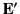is feeding energy into this conductor. The energy is either dissipated as heat, or is used to generate a magnetic field. Ohm's law inside the conductor gives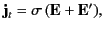(757)

where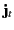is the true current density,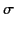is the conductivity, and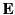is the inductive electric field. Taking the scalar product with, we obtain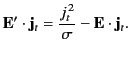(758)

The left-hand side of this equation represents the rate at which the battery does work on the conductor. The first term on the right-hand side is the rate of Joule heating inside the conductor. We tentatively identify the remaining term with the rate at which energy is fed into the magnetic field. If all fields are quasi-stationary (i.e., slowly varying) then the displacement current can be neglected, and Equation (688) reduces to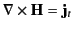. Substituting this expression into Equation (759) and integrating over all space, we get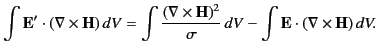(759)

The last term can be integrated by parts using the identity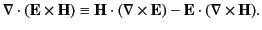(760)

Making use of the divergence theorem, as well as Equation (3), we get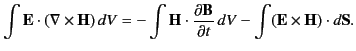(761)

Because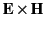falls off at least as fast as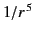in electrostatic and quasi-stationary magnetic fields (comes from electric monopole fields, and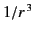from magnetic dipole fields), the surface integral in the previous expression can be neglected. Of course, this is not the case for radiation fields, for whichand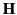fall off like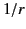. (See Section 1.8.) Thus, the constraint of quasi-stationarity'' effectively means that the fields vary sufficiently slowly that any radiation fields can be neglected.

The total power expended by the battery can now be written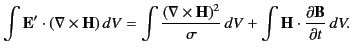(762)

The first term on the right-hand side has already been identified as the energy loss rate due to Joule heating. The last term is obviously the rate at which energy is fed into the magnetic field. The variation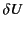in the magnetic field energy can therefore be written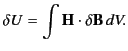(763)

In order to make Equation (764) integrable, we must assume a functional relationship betweenand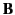. For a medium that magnetizes linearly, the integration can be carried out, in much the same manner as Equation (567), to give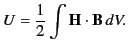(764)

Thus, the magnetostatic energy density inside a linear magnetic material is given by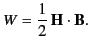(765)

Unfortunately, most interesting magnetic materials, such as ferromagnets, exhibit a nonlinear relationship betweenand. For such materials, Equation (764) can only be integrated between definite states, and the result, in general, depends on the past history of the sample. For ferromagnets, the integral of Equation (764) has a finite, non-zero value whenis integrated around a complete magnetization cycle. This cyclic energy loss is given by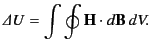(766)

In other words, the energy expended per unit volume when a magnetic material is carried through a magnetization cycle is equal to the area of its hysteresis loop as plotted in a graph of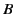against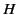. Thus, it is particularly important to ensure that the magnetic used to form transformer cores possess hysteresis loops with comparatively small areas, otherwise the transformers are likely to be extremely inefficient.Next: Exercises Up: Magnetostatics in Magnetic Media Previous: Magnetic Shielding
Richard Fitzpatrick 2014-06-27